Function Repository Resource:

# IntegralApproximationPlot

Compute and plot the approximation to the integral of a function on an interval

Contributed by: Dennis M Schneider
 ResourceFunction["IntegralApproximationPlot"][f,{x,xmin,xmax},method,partition] plots f and an approximation to the integral of f over the interval xmin≤x≤xmax based on the approximation method and the type of the partition.

## Details and Options

The possible values of method are listed below and follow standard mathematical usage, except for possibly "Riemann". When method is set to "Riemann", a random point in each subinterval is selected for the height of the approximating rectangle.
 "Left" the height of the approximating rectangle is determined by the value of the function at the left-hand endpoint of each subinterval "Midpoint" the height of the approximating rectangle is determined by the value of the function at the midpoint of each subinterval "Right" the height of the approximating rectangle is determined by the value of the function at the right-hand endpoint of each subinterval "Upper" the height of the approximating rectangle is determined by the maximum value of the function on each subinterval "Lower" the height of the approximating rectangle is determined by the minimum value of the function on each subinterval "Trap" the approximation is determined by a trapezoid determined by the heights at pairs of endpoints "Simpson" the approximation is determined by quadratic polynomials determined by the endpoints and midpoint of the intervals "Riemann" the height of the approximating rectangle is determined by the value of the function at a random point in each subinterval
Possible partitions are {"Regular",n} for a regular partition with n equal subintervals, {"Maxdx",dx} for a partition with mesh less than dx or an ordered list {x1,x2,,xn} specifying the partition points.
"Exact" is an option for ResourceFunction["IntegralApproximationPlot"] that, if set to True, will return the exact value of the approximation when the partition points are not approximate real numbers.
"DrawGraph" is an option for ResourceFunction["IntegralApproximationPlot"] that, if set to False, will suppress the graph and just return the approximation to the area of the region.
"PolyStyle" is an option for ResourceFunction["IntegralApproximationPlot"] that applies styles to quadratic polynomials used in Simpson’s approximations.
"PrintDisplay" is an option for ResourceFunction["IntegralApproximationPlot"] that, if set to False, will suppress printing of the approximation or the maximum mesh size.

## Examples

### Basic Examples (10)

A left approximation using a regular subdivision with 20 subintervals:

 In:=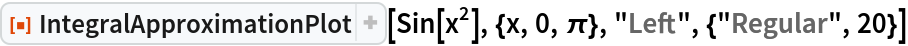Out=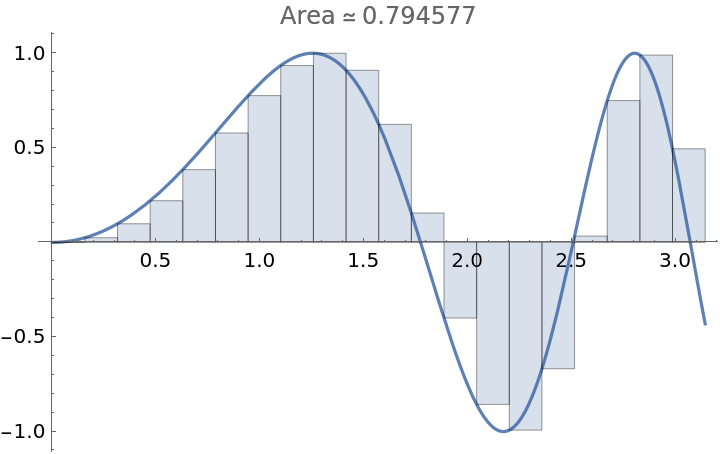An upper approximation using a regular subdivision with 15 subintervals:

 In:=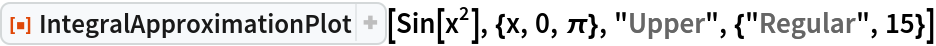Out=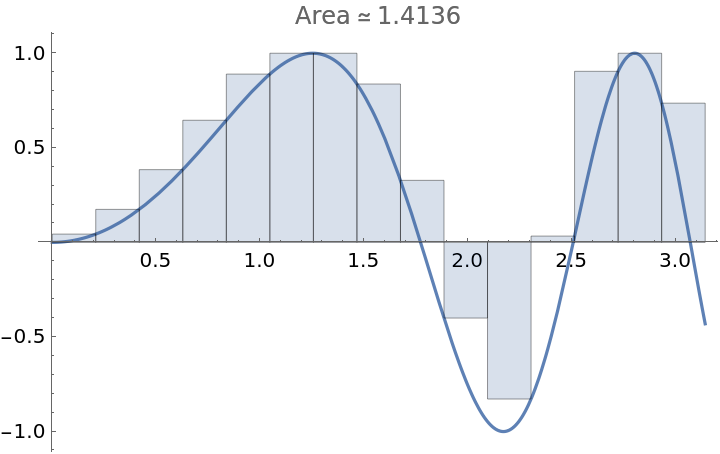Suppress the plot and just return the approximation:

 In:=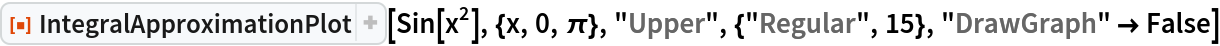Out=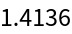Specify the mesh size of the partition:

 In:=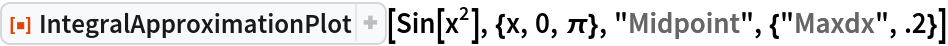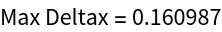Out=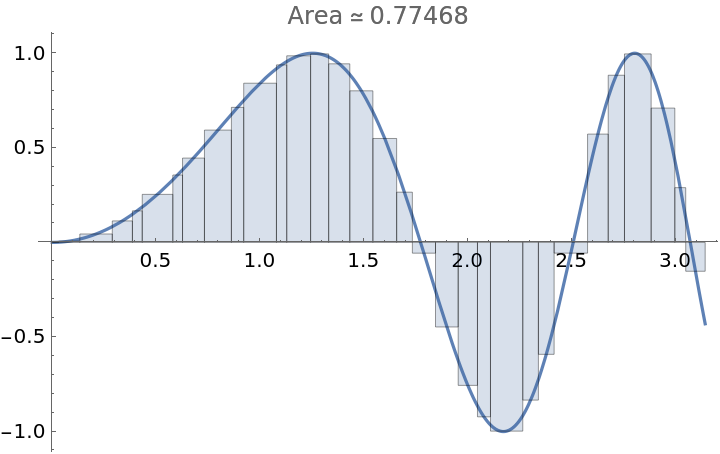The option "Exact" does not apply to partitions containing approximate real numbers:

 In:=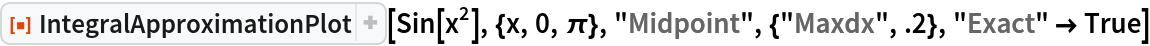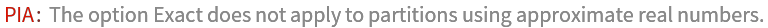Return the exact approximation for a partition using exact numbers:

 In:=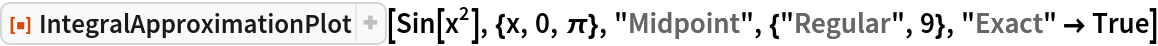Out=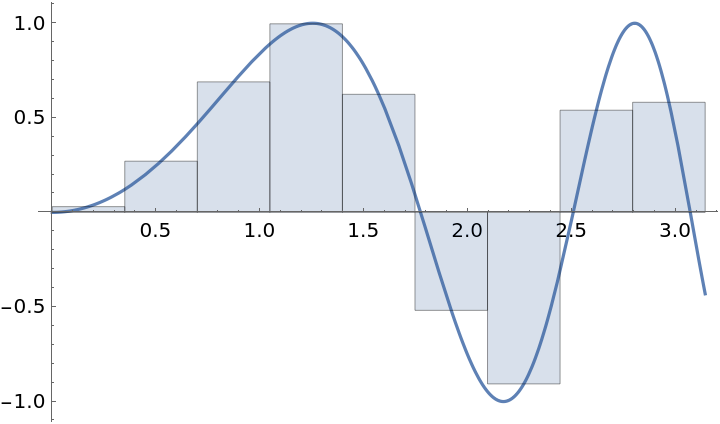Apply a style to the polynomials used in Simpson’s approximation:

 In:=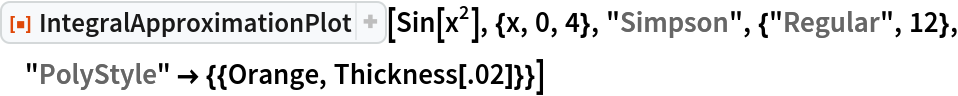Out=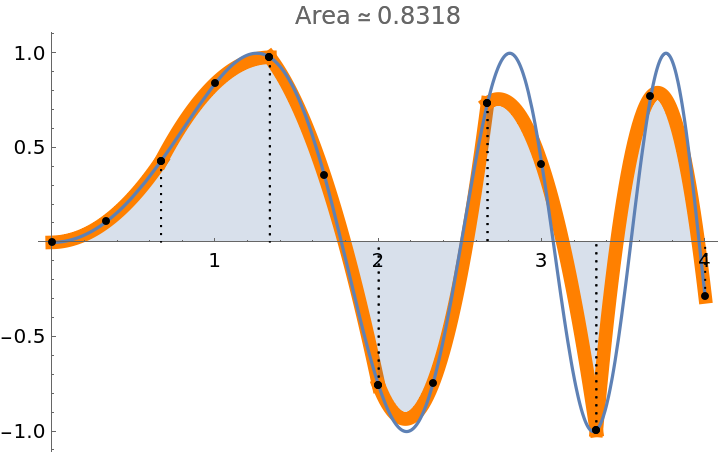Add a style to the filling:

 In:=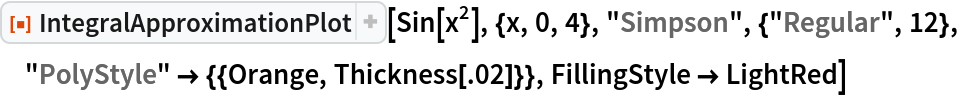Out=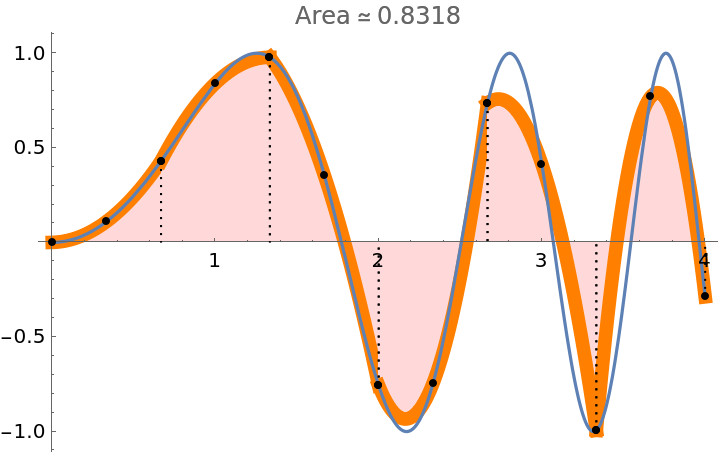Use a user-defined partition:

 In:=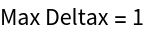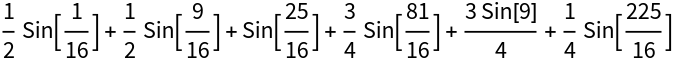Out=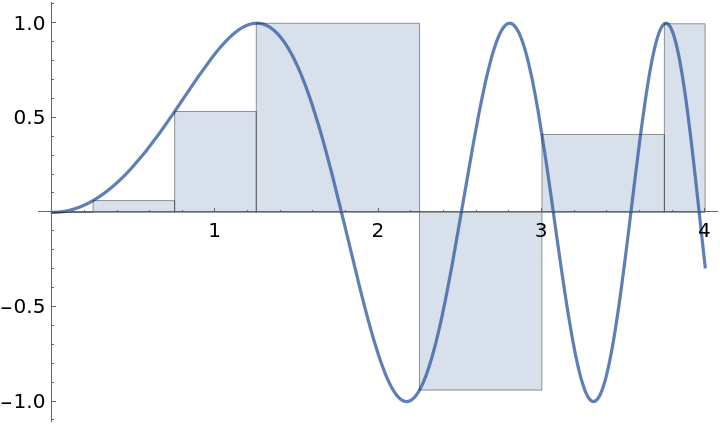In:=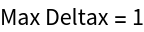Out=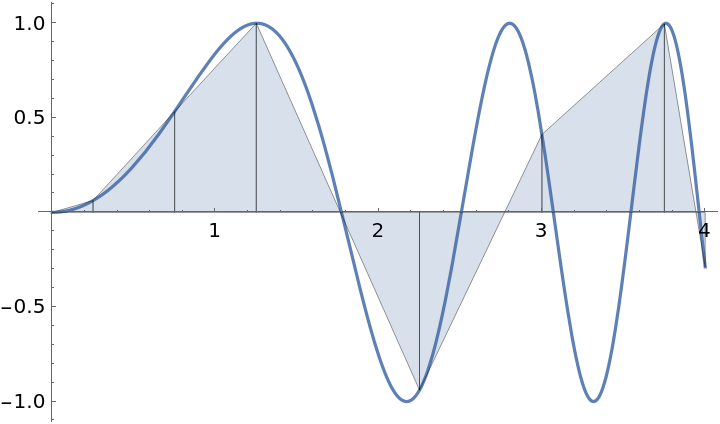Use Manipulate to illustrate all possible combinations of methods and partitions (except user-defined partitions):

 In:=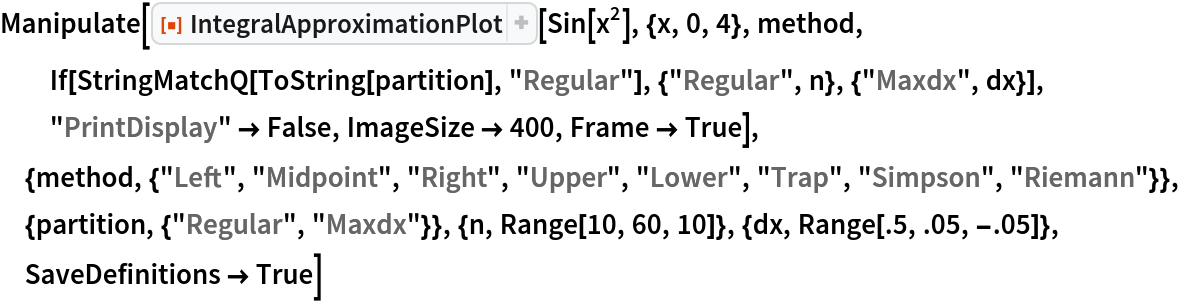Out=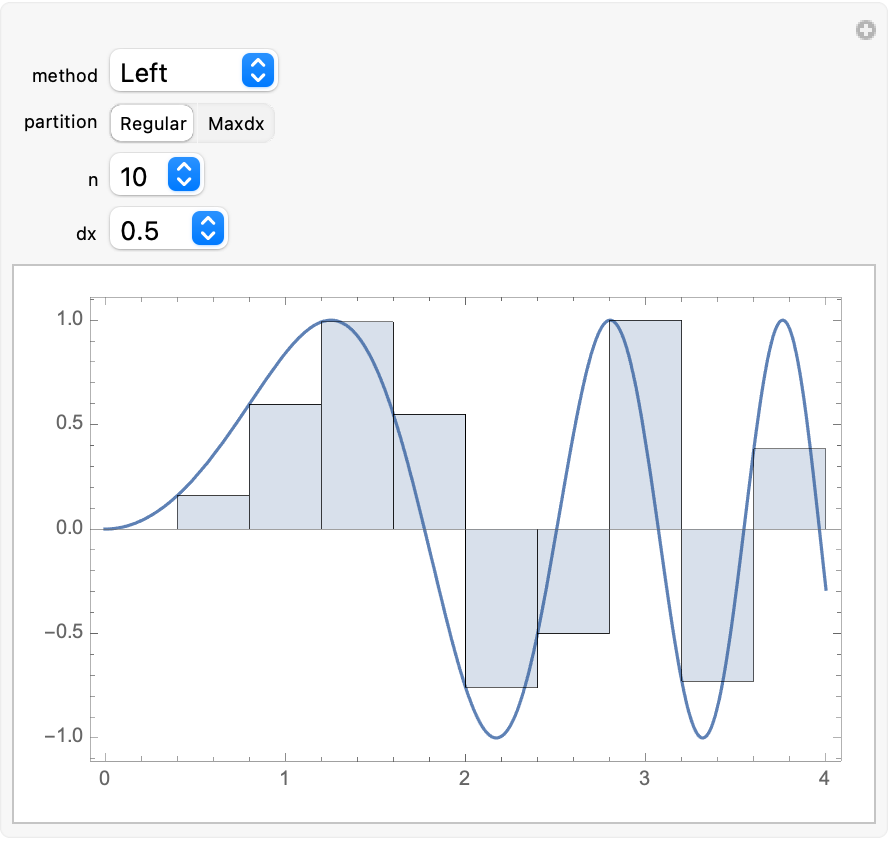### Possible Issues (1)

Simpson’s method will not accept a user-defined partition:

 In:=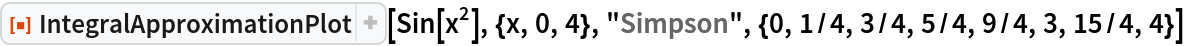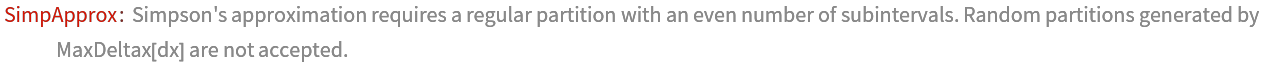## Publisher

Dennis M Schneider

## Version History

• 2.0.0 – 28 August 2019
• 1.0.0 – 02 August 2019